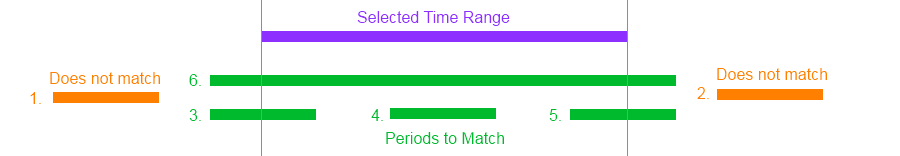# The Overlapping Date Range Test

First posted on September 17, 2013 in Programming

In my line of work, I have to do a lot of comparisons of times and dates. The most common use is something like this:

1. Given a date range selected by the user (say, one week),
2. Get all the periods (from a database or other data source) that overlap with that date range,
3. Where every period has an explicit start and end time.

Simple, right? Well, perhaps not as simple as you might think. I've seen this test performed incorrectly many times, even by experienced programmers. This problem has a very elegant and robust solution, but it is not immediately obvious to most people.

## # A Visualisation of the Problem

When this problem crops up for me (which is quite often), I draw a diagram. Imagine that the selected time range and the periods to match against are drawn as timelines. There are six different situations that can arise:A timeline diagram of the six scenarios of this problem.
1. The period is entirely before the selected range (does not match)
2. The period is entirely after the selected range (does not match)
3. The period starts before the selected range, and ends during the selected range (match)
4. The period starts and ends during the selected range (match)
5. The period starts during the selected range, and ends after the selected range (match)
6. The period starts before the selected range, and ends after the selected range (match)

## # How Not To Do It

First of all, some examples of how not to do it. I pulled these examples from production code, written by experienced programmers. I say this not to point out any lack of skill, but rather how easy it is to do it wrong.

### # Example 1

``````if ((period.Start <= selection.Start && period.Start > selection.End) ||
(period.Start >= selection.Start && period.End <= selection.End) ||
(period.Start < selection.End && period.End >= selection.End))
{
// period overlaps selection
}
``````

### # Example 2

``````var matching = periods.Where(period =>
(period.Start >= selection.Start && period.Start <= selection.End) ||
(period.End >= selection.Start && period.End <= selection.End));
``````

The first thing you might notice about these examples is that they seem rather complex. Both examples get pretty close, but fail to match on one of the conditions from the diagram above. Example 1 fails on scenario 3, and example 2 fails on scenario 6.

## # Deriving the Solution

Both of the examples above fail one test case each, so they could both be resolved by adding a new OR statement to the conditions in each. However, that's not the best solution to this problem. By looking at the problem a bit closer, it's possible to derive a clean solution that's very easy to remember. (Note: For simplicity, I'm going to ignore the case where the times are equal. Changing these examples to and from inclusive/exclusive algorithms is left as an exercise for the reader.)

We start by looking at the six requirements. Since we know that four scenarios must match and two must not, we can build a brute-force solution:

``````if (Condition3 OR Condition4 OR Condition5 OR Condition6)
{
// period overlaps selection
}
``````

Or, in actual code:

``````if ((period.Start < selection.Start && period.End > selection.Start) ||
(period.Start > selection.Start && period.End < selection.End) ||
(period.Start < selection.End && period.End > selection.End) ||
(period.Start < selection.Start && period.End > selection.End))
{
// period overlaps selection
}
``````

This code should work and will pass all tests - however, it's complex and unwieldy. I cannot say for sure that it doesn't have any bugs without running unit tests over it, and I triple-checked it after writing it just now. It's not very nice code, so we should try to optimise it if we can.

Let's look at this a different way. If we have six scenarios and 4 are valid, then the other 2 are invalid. So, instead of explicitly looking for the valid situations, we can instead get rid of the invalid ones. Like so:

``````if (NOT(Condition1 OR Condition2))
{
// period overlaps selection
}
``````

Implemented in code:

``````if (!(
(period.Start < selection.Start && period.End < selection.Start) ||
(period.Start > selection.End && period.End > selection.End))
)
{
// period overlaps selection
}
``````

This is better, but can be improved. Since we know that a period start is always before the period end, we can exclude the extra comparisons:

``````if (!(period.End < selection.Start || period.Start > selection.End))
{
// period overlaps selection
}
``````

This is starting to look much better, and one small change simplifies it further. Since binary logic states that NOT(A OR B) is equivalent to NOT(A) AND NOT(B), we can make this change:

``````if (period.End > selection.Start && period.Start < selection.End)
{
// period overlaps selection
}
``````

...and now we have a simple solution that covers all use cases. The code is easy to maintain and read. It also lets us explain the algorithm in clear English: A period overlaps a selected date range when it ends after the selected start date and starts before the selected end date. As an added bonus, this method works for any sortable value, not just dates.